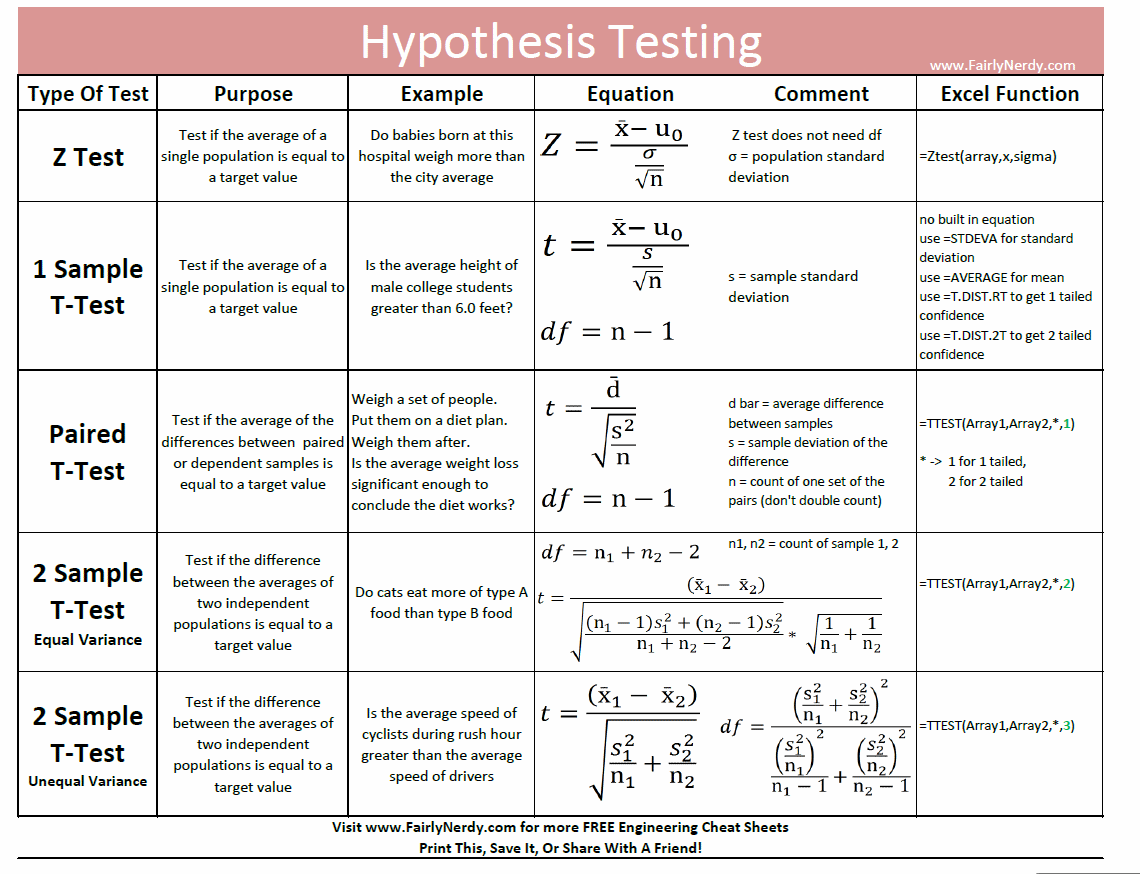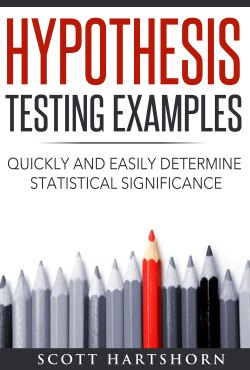# Understanding Statistical Significance

## Statistical Significance in Real Life

Statistical significance is a way of quantifying how unlikely something that you are measuring is, given what you know about the baseline.   Exactly how unlikely something needs to be before it is statistically significantly depends on the context.  You likely have an intuitive understanding of statistical significance based on your own life.

For instance,  if you were at a United States airport, and it was announced that your plane was 15 minutes late, you wouldn’t think that it was anything unusual.  But if you were at a Japanese bullet train station, and found out that it was going to be 15 minutes late, you would probably think that was at least somewhat odd.

Why does one seem like a more significant event than the other ?   It is because you know that planes are frequently late, where the trains almost never are.   So the trains being late is more significant because it is more different than the normal day to day variation than the plane being late.

## Plot The Delay

Statistical Significance is very easy to understand on a probability density plot.   The red line shows 15 minutes late.   The blue line shows how likely a train will be any given time late, and the green line shows how likely a plane will be any given time late.   The total area under each of the blue and green lines is 1

It is clear on the chart that very few trains are more than 15 minutes late, but  a lot of planes are.There are really two things going on in the chart.  The first is that the average plane is more late than the average train is.   The average plane is 10 minutes late, and the average train is 0 minutes late.   So being 15 minutes late is bigger difference from average than for a train than a plane.

The second thing that is going on is that the distribution of plane lateness is a lot wider than the distribution of train lateness.  There is a lot more variation in the plane departure time than there is in the train departure time.   Because of that the plane lateness would have to be even greater to be unusual

## The Gist of Statistical Significance

Statistical Significance means quantifying the probability of how unlikely an event is.  Exactly what is statistically significant depends on context, but typical numbers considered statistically significant are if something would have less than a 5% chance, less than a 1% chance, or less than a .5% chance of occurring if there wasn’t some difference between what you are measuring and the baseline.

The information that is important to statistical significance are

• How many measurements you have – The more measurements you have, the more likely you have measured the full population of what is occurring, and not just a non-representative sample
• How different the average of your measurements is from the expected average  –  The bigger the difference, the more likely it is significant.
• How much variation there is in the measurements.  –   The less variation there is in the measurements, i.e. the tighter the spread is, the smaller the difference needs to be to be significant

There are small differences in the equations based on exactly what has been measured, but essentially all of the equations boil down to

• Get a number which is the difference in average values, multiplied by the square root of the number of measurements you have, and divided by the square root of the variation in your measures.   Call that number the “Test Statistic”
• The larger the Test Statistic the more statistically significant the difference.
• Look up the Test Statistic in the appropriate “Z-Table” or “T-Table” to find the probability that there is a statistically significant difference between your samples, as opposed to just random variation

## Equations For Statistical Significance

Now that you have a general understanding of Statistical Significance, it is time to look at the equations.   The most commonly used test for statistical significance is the Z-Test.   You use this test if you have a lot of measurements  (at least 20, preferably at least 40) and you are comparing it against a population with known values.   For example, you would use this test if you work at a hospital that had 500 babies born in it the past year, and you wanted to see if the average weight of those babies was different than the average weight of every baby born in your city.Where

• X_bar   : is the average of the measured data
• U_0      : is the population average
• Sigma  : is the population standard deviation
• n           : is the number of measured samples

You then look up the Z-value in a Z-Table to get probability

There are a few other different equations for Statistical significance called “T-Tests”.   You would use one of these T-Tests instead of a Z-test for one of these reasons

• The number of measurements you have is small, certainly you would use a T-Test with fewer than 20 measurements, or maybe fewer than 50
• You want to compare before and after measurements for the same individual.  For instance, if you have a before and after measurement for 20 people after a diet, you would use a certain type of T-Test.

## What is the difference between a Z-Test and a T-Test?

What is a T-test vs a Z-test, and how do you know when to use a Z-test or a T-test?  The thing to understand about T-Tests, is that they are almost the same as the Z-Test, and will give almost the same answer as you increase the number of measurements that you have.  The whole point of T-Tests is that they put more area at the tail of the normal curve, instead of the middle to account for uncertainty you would have in your measured mean and standard deviation if you have a very small sample size.   Once you get above 20 or so measurements the difference between Z-test results and T-test results becomes vanishingly small.

The plots below show the probability density for a Z-curve, and T-test curves with different sample sizesOnce you get past 20 or so measurements (green line, hardly visible) there really isn’t much of a difference between a T-Test or a Z-test (purple line).  However if you only have a few measurements than the T-Test results will need a lot greater Test Statistic to give a statistically significant result

It can be a little bit confusing knowing exactly which test to use, but using the exact right test isn’t that important unless you are taking an exam or righting a scientific paper.  The tests will all give similar results assuming you have more than 10 measurements, and very similar assuming you have 30 or more.

For a better understanding of the different types of tests, you can refer to this cheat sheet I put together giving the formulas for each test, and when they are used.## Examples of Z-Test vs T-Tests

This post was intending to give an intuitive understanding of statistical significance.   If you are interested in looking at examples of Z-Tests and T-tests and exactly how they are used and in what circumstance you might use one or the other, you can find some examples in this book I’ve put on AmazonOr you can get an Excel file with different hypothesis testing examples here.